# Brocard point

(diff) ← Older revision | Latest revision (diff) | Newer revision → (diff)

The first (or positive) Brocard point of a plane trianglewith vertices,,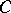is the interior pointoffor which the three angles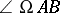,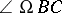,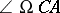are equal. Their common valueis the Brocard angle of.

The second (or negative) Brocard point of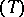is the interior pointfor which. Their common value is again. The Brocard angle satisfies. The two Brocard points are isogonal conjugates (cf. Isogonal); they coincide ifis equilateral, in which case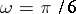.

The Brocard configuration (for an extensive account see [a6]), named after H. Brocard who first published about it around 1875, belongs to triangle geometry, a subbranch of Euclidean geometry that thrived in the last quarter of the nineteenth century to fade away again in the first quarter of the twentieth century. A brief historical account is given in [a5].

Although his name is generally associated with the pointsand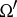, Brocard was not the first person to investigate their properties; in 1816, long before Brocard wrote about them, they were mentioned by A.L. Crelle in [a4] (see also [a8] and [a11]). Information on Brocard's life can be found in [a7].

The Brocard points and Brocard angle have many remarkable properties. Some characteristics of the Brocard configuration are given below.

Letbe an arbitrary plane triangle with vertices,,and angles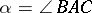,,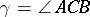. If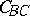denotes the circle that is tangent to the line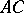atand passes through the verticesand, thenalso passes through. Similarly for the circlesand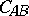. So the three circles,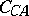,intersect in the first Brocard point. Analogously, the circle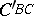that passes throughandand is tangent to the line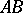at, meets the circles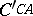and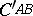in the second Brocard point. Further, the circumcentre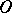ofand the two Brocard points are vertices of a isosceles triangle for which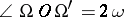. The lengths of the sides of this triangle can be expressed in terms of the radius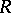of the circumcircle of, and the Brocard angle:The Brocard circle is the circle passing through the two Brocard points and. The Lemoine pointof, named after E. Lemoine, is a distinguished point of this circle, and the length of the line segment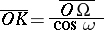gives the diameter of the Brocard circle.

The Brocard angleis related to the three angles,,by the following trigonometric identities: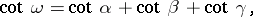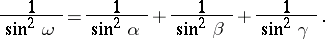Due to a remarkable conjecture by P. Yff in 1963 (see [a14]), modest interest in the Brocard configuration arose again during the 1960s, 1970s and 1980s. This conjecture, known as Yff's inequality,is unusual in the sense that it contains the angles proper instead of their trigonometric function values (as could be expected). A proof for this conjecture was found by F. Abi-Khuzam in 1974 (see [a2]). In [a12] and [a1] a few inequalities of similar type were proposed and subsequently proven.

How to Cite This Entry:
Brocard point. Encyclopedia of Mathematics. URL: http://encyclopediaofmath.org/index.php?title=Brocard_point&oldid=11736
This article was adapted from an original article by R.J. Stroeker (originator), which appeared in Encyclopedia of Mathematics - ISBN 1402006098. See original article